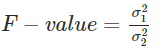Request a Tool

F Test Calculator

F Test Calculator is use to calculate F value from two data set values.

F-VALUE
0

Formula• F-value = F-value of F-test
• a1 = Variance of data set 1
• a2 = Variance of data set 2

Defination / Uses

Under the null hypothesis, an F-test is any statistical test in which the test statistic has an F-distribution. It's most commonly used when comparing statistical models that have been fitted to a data set to determine which model better fits the population from which the data were sampled.

An F-Test is a test that uses the F-distribution, which was named after Sir Ronald Fisher. The Fischer-Snedecor distribution, sometimes known as the F-distribution, is a continuous statistical distribution used to see if two observed samples have the same variance. If a variable's distribution takes the shape of an F-curve, it is said to have an F-distribution. The illustration below shows some examples of F-curves. For evaluating two population variances, the F-distribution has two degrees of freedom, or the number of independent pieces of information for each population.